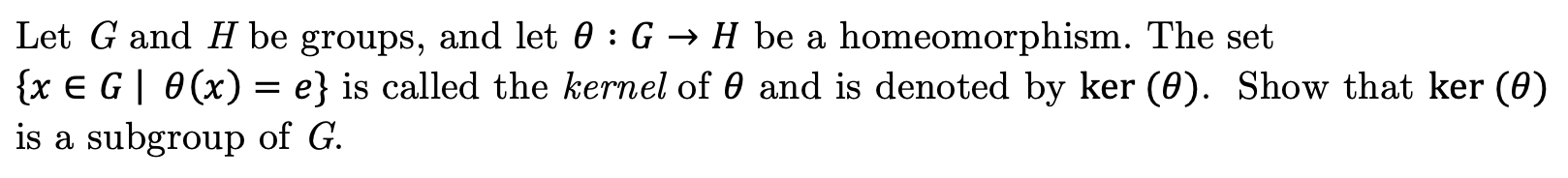# Let G and H be groups, and let 0 : G -» H be a{x E G 0(x) = e} is called the kernel of 0 and is denoted by ker (0). Show that ker (e)is a subgroup of Ghomeomorphism. The set

Question
42 viewshelp_outlineImage TranscriptioncloseLet G and H be groups, and let 0 : G -» H be a {x E G 0(x) = e} is called the kernel of 0 and is denoted by ker (0). Show that ker (e) is a subgroup of G homeomorphism. The set fullscreen
check_circle

Step 1

From the given information:

Let G and H be the groups

Step 2

It is needed to show that:

Step 3

To show it to be a subgroup use one...

### Want to see the full answer?

See Solution

#### Want to see this answer and more?

Solutions are written by subject experts who are available 24/7. Questions are typically answered within 1 hour.*

See Solution
*Response times may vary by subject and question.
Tagged in

### Math